# Kraken Daily Market Report for September 09 2020

## Overview

• Total trading at $208.1 million, the lowest it has been in 10 days as Bitcoin was relatively calm and was within +/- 1% all day. • Ethereum reversed some of its losses from the day before and rose steadily over the day to close +4.2% over USD. • Most cryptos were positive over USD (Tron -3.3% and Storj -1.8% the exceptions). Big returns from Polkadot (+10%) and Algo (+18%). • EUR trading volume (50%) beat out USD (47%), and EUR/USD was the 10th most traded pair. September 09, 2020$208.1M traded across all markets today
Crypto, EUR, USD, JPY, CAD, GBP, CHF, AUD
XBT
$10230. ↑1.1%$97.5M
ETH
$351.03 ↑4.2%$49.8M
USDT
$1.0000 ↑0.0%$25.8M
$12.361 ↑5.4%$8.05M
DOT
$4.7001 ↑10%$7.66M
XRP
$0.2389 ↑1.0%$3.67M
USDC
$0.9999 ↓0.03%$2.75M
ALGO
$0.4130 ↑18%$1.94M
XTZ
$2.5319 ↑0.9%$1.88M
TRX
$0.0339 ↓3.3%$1.83M
BCH
$224.20 ↑0.8%$1.71M
LTC
$47.835 ↑0.8%$1.33M
OXT
$0.3461 ↑2.3%$1.21M
$0.0933 ↑2.6%$1.17M
XMR
$84.229 ↑0.22%$966K
DAI
$1.0295 ↑0.5%$938K
OMG
$3.8536 ↑9.3%$805K
ATOM
$5.3274 ↑7.7%$796K
GNO
$60.665 ↑7.3%$679K
KAVA
$2.4773 ↑7.9%$624K
EOS
$2.7528 ↓0.4%$563K
COMP
$178.03 ↑2.5%$423K
WAVES
$2.3985 ↑3.5%$422K
ETC
$5.0785 ↓0.31%$395K
REP
$17.229 ↑7.9%$357K
XLM
$0.0782 ↑1.2%$356K
DASH
$75.609 ↑1.2%$331K
BAT
$0.2567 ↑2.3%$314K
MLN
$45.216 ↑3.6%$311K
PAXG
$1954.8 ↑0.9%$293K
KNC
$1.2197 ↑3.4%$286K
ZEC
$59.965 ↑2.3%$262K
QTUM
$2.4410 ↑3.6%$235K
STORJ
$0.4693 ↓1.8%$187K
XDG
$0.0027 ↑1.2%$184K
NANO
$0.8382 ↑2.7%$132K
SC
$0.0033 ↑5.6%$122K
ICX
$0.4197 ↑4.3%$114K
LSK
$1.4046 ↑5.2%$111K
REPV2
$16.532 ↑7.6%$53.0K

#####################. Trading Volume by Asset. ##########################################

The figures below break down the trading volume of the largest, mid-size, and smallest assets. Cryptos are in purple, fiats are in blue. For each asset, the chart contains the daily trading volume in USD, and the percentage of the total trading volume. The percentages for fiats and cryptos are treated separately, so that they both add up to 100%.

Figure 1: Largest trading assets: trading volume (measured in USD) and its percentage of the total trading volume (September 09 2020)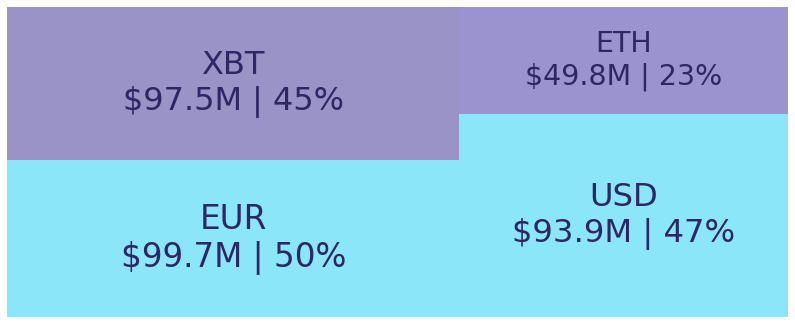Figure 2: Mid-size trading assets: (measured in USD) (September 09 2020)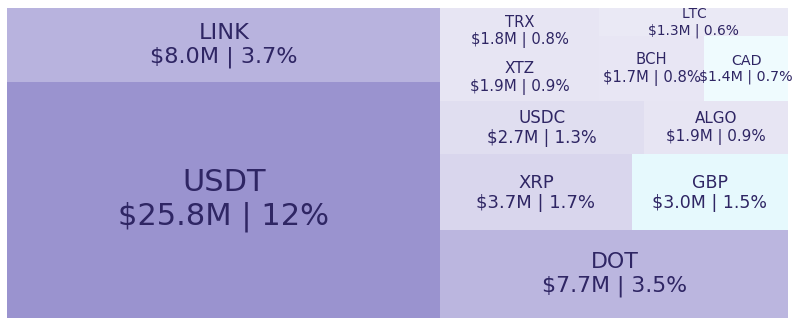Figure 3: Smallest trading assets: (measured in USD) (September 09 2020)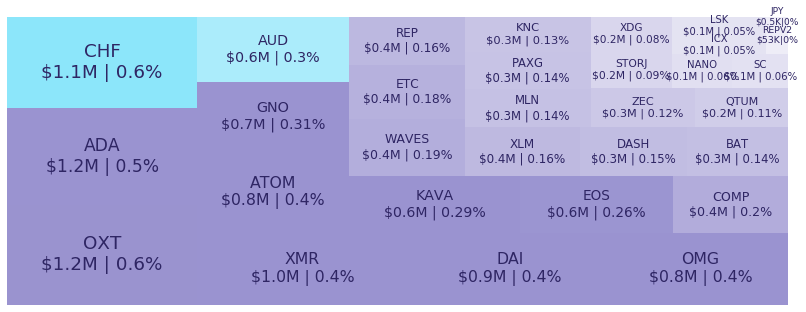Spread percentage is the width of the bid/ask spread divided by the bid/ask midpoint. The values are generated by taking the median spread percentage over each minute, then the average of the medians over the day.

Figure 4: Average spread % by pair (September 09 2020)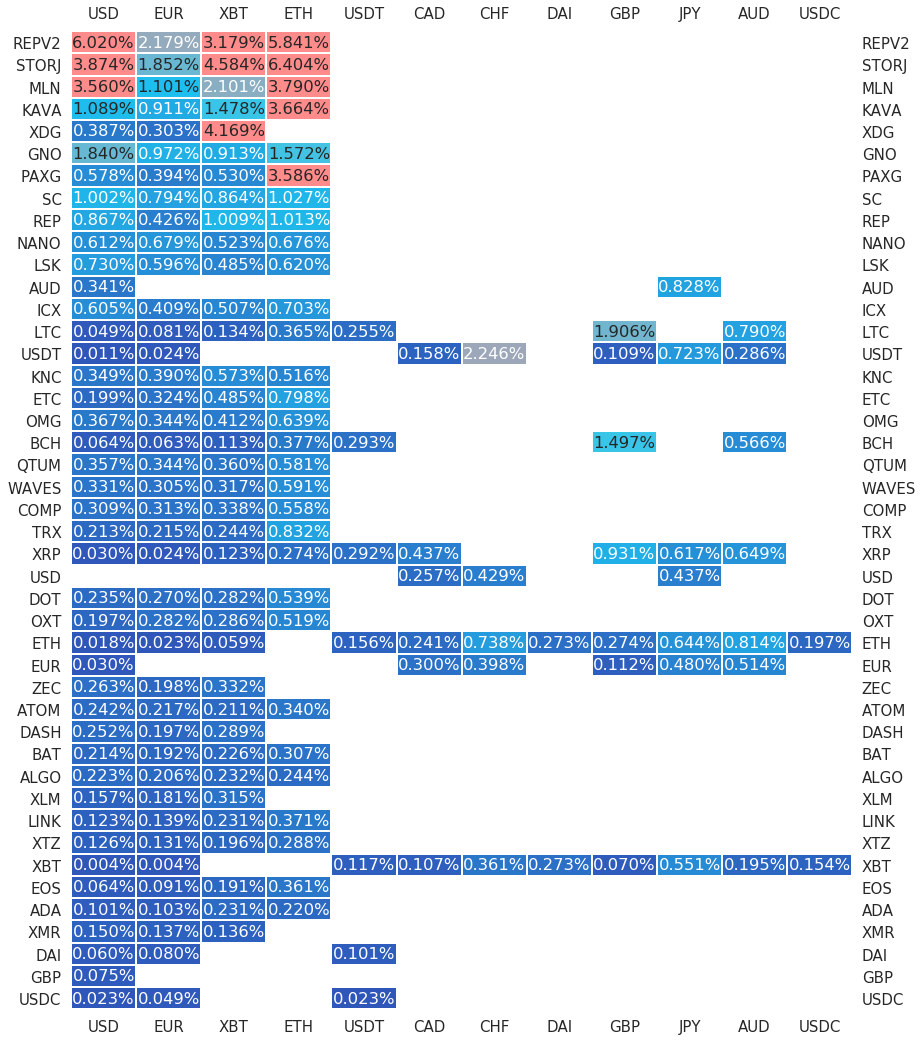.

#########. Returns and Volume ############################################

## Returns and Volume

Figure 5: Returns of the four highest volume pairs (September 09 2020)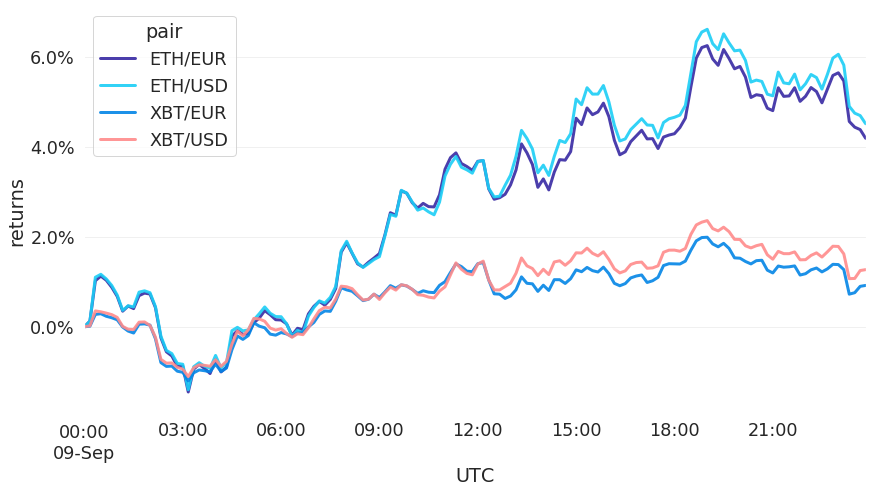Figure 6: Volume of the major currencies and an average line that fits the data to a sinusoidal curve to show the daily volume highs and lows (September 09 2020)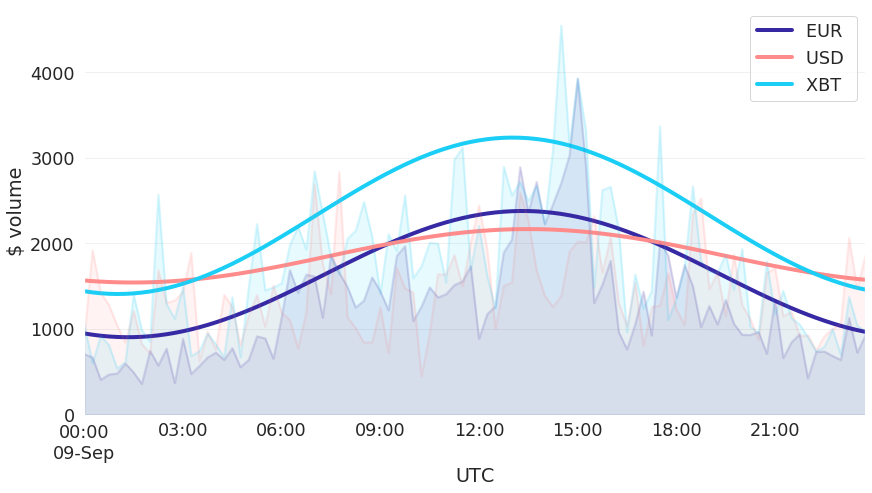###########. Daily Returns. #################################################

## Daily Returns %

Figure 7: Returns over USD and XBT. Relative volume and return size is indicated by the size of the font. (September 09 2020)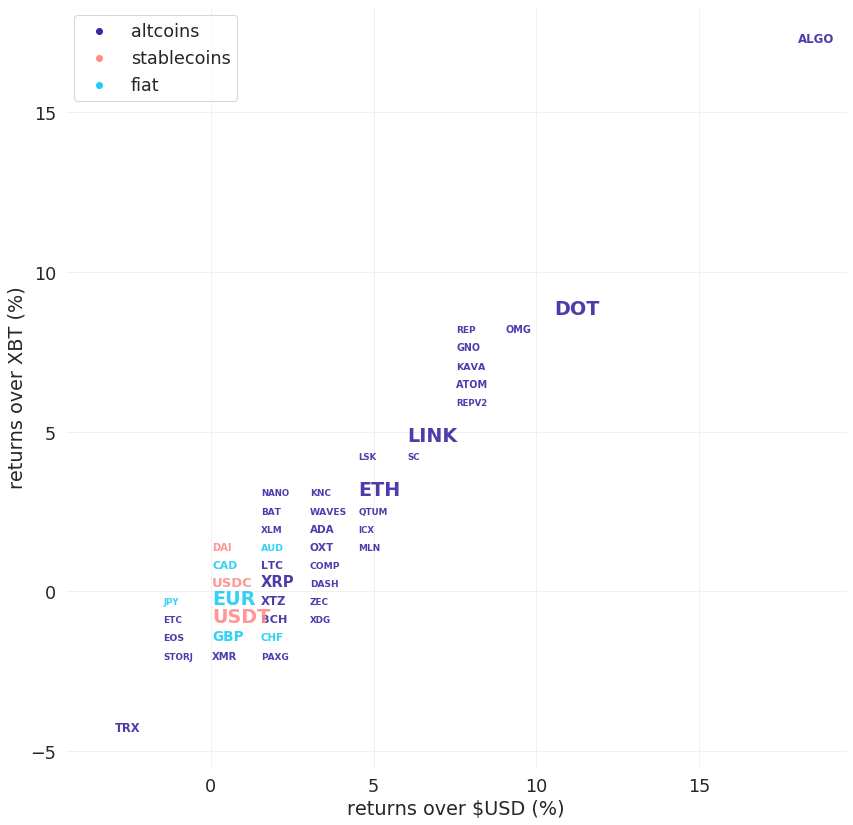###########. Disclaimer #################################################

The values generated in this report are from public market data distributed from Kraken WebSockets api. The total volumes and returns are calculated over the reporting day using UTC time.